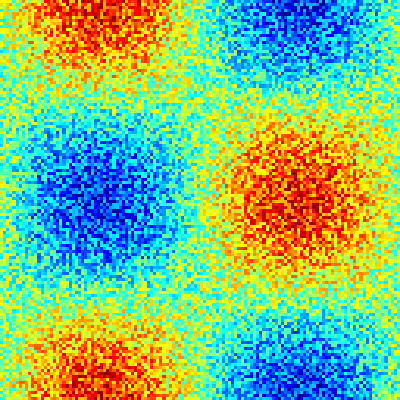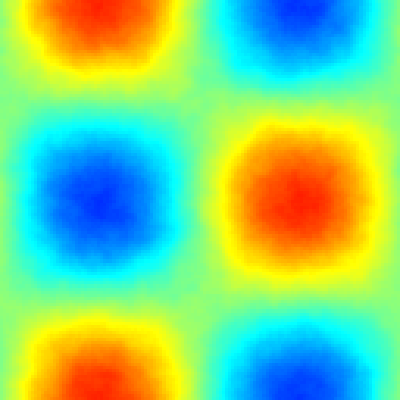# The Helmholtz filter

Reading the work of Fodor et al. (2019), I learned about the Helmholtz filter (Foias et al, 2001): An original field \phi, may be filtered (\phi_f) with filter length scale \Delta, according to:

\displaystyle \left(1 - \left(\frac{\Delta}{2\pi}\right)^2\nabla^2\right) \phi_f = \phi.

A neat feature is that \phi_f may be subjected to the same boundary conditions as \phi, such that the filtered field could enherit crucial properties of the original fields (i.e. assuming a solution even exists).

We solve the equation with the Poisson-Helmholtz solver and visualize the result with the output_ppm() utility.

#include "poisson.h"
#include "utils.h"

#define DELTA 1.0

scalar phi[], phif[];

int Helmholtz_filter (scalar phi, scalar phif, double Delta) {
double sq_alpha = -sq(Delta/(2.*pi));
const face vector alphaf[] = {sq_alpha, sq_alpha};
return poisson (phif, phi, alphaf, unity).i;
}

int main() {
L0 = 2*pi;
foreach_dimension()
periodic (left);
init_grid (128);
foreach()
phi[] = sin(x) * cos(y) + 0.5*noise();
output_ppm (phi, file = "phi.png", n = 400,
min = -1.4, max = 1.4);The original field (\phi)

  Helmholtz_filter (phi, phif, DELTA);
output_ppm (phif, file = "phif.png", n = 400,
min = -1.4, max = 1.4);

The result for \phi_f:The filtered field (\phi_f)

Looks good..

Recall the Helmholtz filter

\displaystyle \left(1 - \left(\frac{\Delta}{2\pi}\right)^2\nabla^2\right) \phi_f = \phi.

One may rewrite it as

\displaystyle \left(\frac{\Delta}{2\pi}\right)^2\nabla^2 \phi_f - \phi_f = -\phi, \displaystyle \mathrm{or\ as }\rightarrow \displaystyle \phi_f - \phi = \left(\frac{\Delta}{2\pi}\right)^2\nabla^2 \phi_f.

From which we recognize the structure of the backward-Euler discretization for the diffusion equation:

\displaystyle \frac{\phi^{n+1} - \phi^{n}}{\mathrm{d}t} = D\nabla^2\phi^{n+1},

where \phi^{n+1} \leftarrow \phi_f, \phi^n \leftarrow \phi, and \mathrm{d}t \times D \leftarrow \left(\frac{\Delta}{2\pi}\right)^2.

Fodor K, Mellado, JP, Wilczek M (2019) On the role of large-scale updrafts and downdrafts in deviations form Monin-Obukhov similarity theory in free convection. Boundary Layer Meteorology. DOI

Foias C, Holm D, Titi E (2001) The Navier–Stokes-alpha model of fluid turbulence. Physica D 152–153:505–519

}